### Home > A2C > Chapter 13 > Lesson 13.1.4 > Problem13-76

13-76.
1. Parts (a) through (d) of problem 13-75 represent a general pattern known as the sum and difference of cubes. Use this pattern to factor each of the following polynomials. Homework Help ✎

1. x3 + y3

2. x3 − 27

3. 8x3y3

4. x3 + 1

5. Make up another problem involving the sum or difference of cubes and show how to factor it.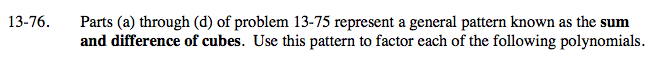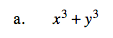See problem 13-75.

(x + y)(x2xy + y2)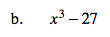See part (a).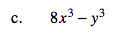See part (a).

(2xy)(4x2 + 2xy + y2)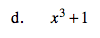See part (a).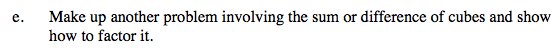Substitute values into the expression in part (a). Then factor as in part (a).